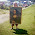Meaningful Math

Sunday, January 20, 2013 1 Comments

Last Thursday I was doing some classroom visits with Nipmuc Principal John Clements and we couldn't help but notice a true conversation piece that was erected in the library media center.  It looked like this:

Standing 8 feet tall, it certainly was an impressive sight.  Math teacher Rob Messick and his two classes of sophomores in Fundamentals of Geometry constructed this 3-D figure, known as Sierpinski's pyramid, over the past week and a half.  They carefully cut thousands of equilateral triangles and used plenty of scotch tape to construct the repeating geometric pattern of a tetrahedral (4-sided) pyramid.

When Mr. Clements and I went into the library the students were admiring the fruition of their efforts.  They enthusiastically answered questions about the pyramid, sharing with us the fact that the figure was perfectly symmetrical and that the base pattern kept repeating in an exponential fashion (each unit referred to as a "stage").  The students were able to tell that this figure had five stages and if they were to construct a sixth stage, it would be 32 feet in height.  They shared that if this pyramid was 100 stages, it would be thousands of light years tall. They were also able to cite the volume of open versus closed spaces in the figure based open the volumes of each successive stage they created.

It turns out that Sierpinski's pyramid contains plenty of higher level mathematical concepts, including tessellations, fractals, exponential magnitude, and symmetry to name just a few.  These concepts clearly speak to higher order thinking, i.e., where students must analyze, predict, integrate, and synthesize difficult content so it makes sense.  Here is a animated visual showing the repeating various stages Sierpinski's triangle:

To put it in perspective, here is the mathematical algorithm that goes with this repeating pattern:

The area of the Sierpinski Triangle approaches 0. This is because with every iteration 1/4 of the area is taken away. After an infinit number of iterations the remaining area is 0.

The number of triangles in the Sierpinski triangle can be calculated with the formula:

N=3^k - 1

Where n is the number of triangles and k is the number of iterations.

Okay, not the absolute most difficult math here... but without a visual cue, probably not the most fascinating either.  What Mr. Messick was successfully able to facilitate was true project-based learning for his students.  He gave them a challenge (they had no idea what the final outcome of their construction would be) and allowed them to discover the mathematical concepts as they constructed the figure, guiding their hypotheses as they progressed.  This type of learning is the most impactful for students because they will truly understand because they are engaged in hands-on learning.  As the Chinese proverb states:

I hear and I forget
I see and I remember
I do and I understand

I am confident that Mr. Messick's understand this content and will not forget this project for some time!